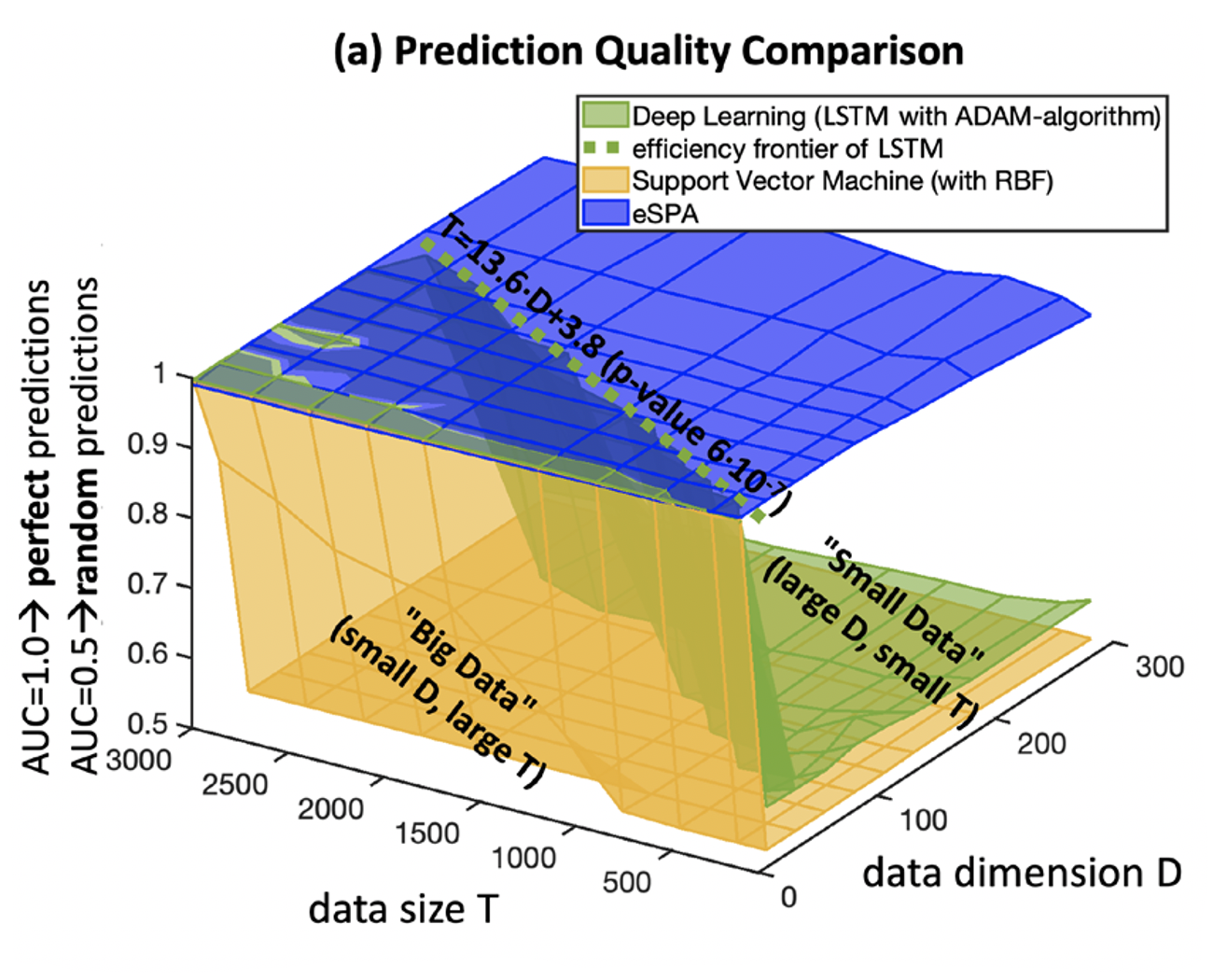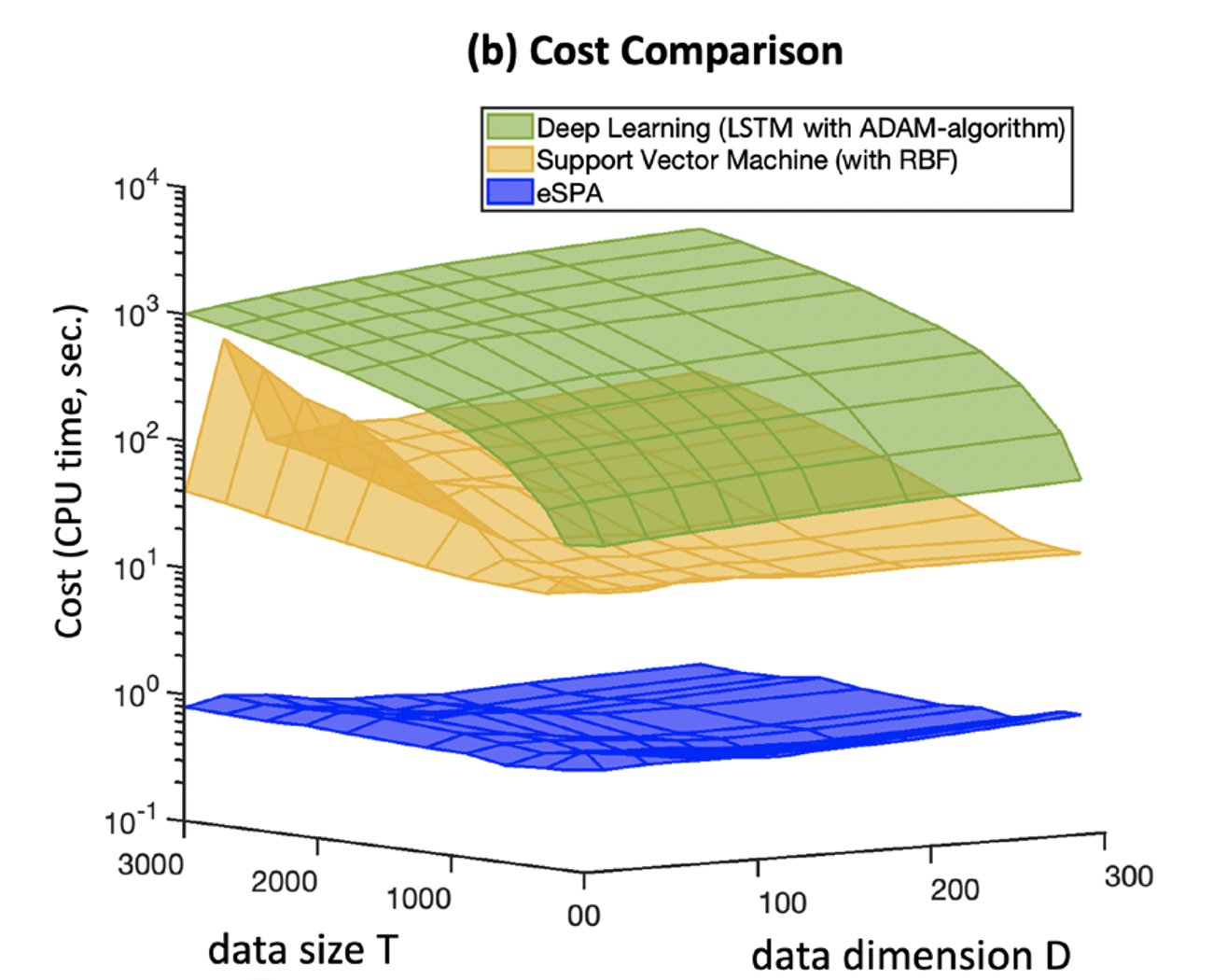# Research

Central goal of the research group is to develop computationally-scalable and mathematically-justified methodologies for “small sample grey-box learning” combined with big data analysis challenges, enabling to obtain valid results even by learning from a few examples only. In recent years, the small data challenges emerging in various applications, particularly in biomedicine, in geosciences and in economics/finance, have indicated an urgent need for replacing the current state-of-the-art data-hungry AI and ML tools with algorithms that can smartly handle available information and are still statistically valid with fewer data. The group of Illia Horenko develops several “grey-box” small data analysis algorithms on the boundary between ML and applied mathematics, based on combinations of Hidden Markov Models with dimension reduction and stochastic differential equations. The research group is advancing these algorithms with data sets from different disciplines, including biomedicine, finance and geosciences. In contrast to the state-of-the-art in ML, these methods do not solve distinct data analysis steps sequentially in a pipeline but solve all of these problems jointly and simultaneously based on a scalable numerical solution of the appropriate optimal discretization problem. They allow obtaining geometrically-interpretable models trained with numerical optimization algorithms with linear computational cost scaling. Furthermore, these methods are characterized by mathematically-justified regularity and optimality of the obtained solutions and a parallel communication cost proven to be independent of the sample statistics size.(a) Overfitting boundaries of DL and SVM in small data learning problem.(b) Computational costs of DL and Support Vector Machines increase polynomial with statistics versus linear cost scaling in eSPA

Figure (small data learning challenge): deep learning(DL) and Support Vector Machine with radial basis functions (SVM) compared to the Entropy-based Scalable Probabilistic Approximation Algorithm (eSPA from I.Horenko, Neural Computation, 32(8):1563-1579, 2020).